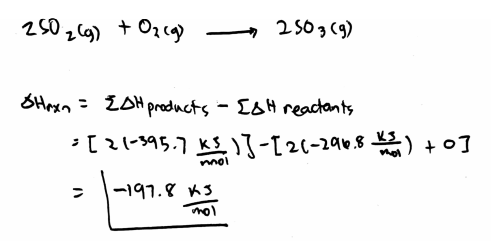# Problem: Sulfur dioxide, SO2(g), can react with oxygen to produce sulfur trioxide, SO3(g), by the following reaction 2SO2(g) + O2(g) → 2SO3(g) The standard enthalpies of formation for SO2(g) and SO3(g) are ΔH°f [SO2(g)] = -296.8 kJ/mol ΔH°f [SO3(g)] = -395.7 kJ/mol Calculate the amount of energy in the form of heat (kJ) that is produced when a volume of 3.24 L of SO2(g) is converted to 3.24 L of SO3(g) according to this process at a constant pressure and temperature of 1.00 atm and 25.0°C. Assume ideal gas behavior.

###### FREE Expert Solution
79% (238 ratings)
###### FREE Expert Solution79% (238 ratings)###### Problem Details

Sulfur dioxide, SO2(g), can react with oxygen to produce sulfur trioxide, SO3(g), by the following reaction

2SO2(g) + O2(g) → 2SO3(g)

The standard enthalpies of formation for SO2(g) and SO3(g) are

ΔH°[SO2(g)] = -296.8 kJ/mol

ΔH°[SO3(g)] = -395.7 kJ/mol

Calculate the amount of energy in the form of heat (kJ) that is produced when a volume of 3.24 L of SO2(g) is converted to 3.24 L of SO3(g) according to this process at a constant pressure and temperature of 1.00 atm and 25.0°C. Assume ideal gas behavior.

What scientific concept do you need to know in order to solve this problem?

Our tutors have indicated that to solve this problem you will need to apply the Enthalpy of Formation concept. You can view video lessons to learn Enthalpy of Formation. Or if you need more Enthalpy of Formation practice, you can also practice Enthalpy of Formation practice problems.

What is the difficulty of this problem?

Our tutors rated the difficulty ofSulfur dioxide, SO2(g), can react with oxygen to produce sul...as medium difficulty.

How long does this problem take to solve?

Our expert Chemistry tutor, Sabrina took 8 minutes and 10 seconds to solve this problem. You can follow their steps in the video explanation above.

What professor is this problem relevant for?

Based on our data, we think this problem is relevant for Professor Marohn's class at CORNELL.